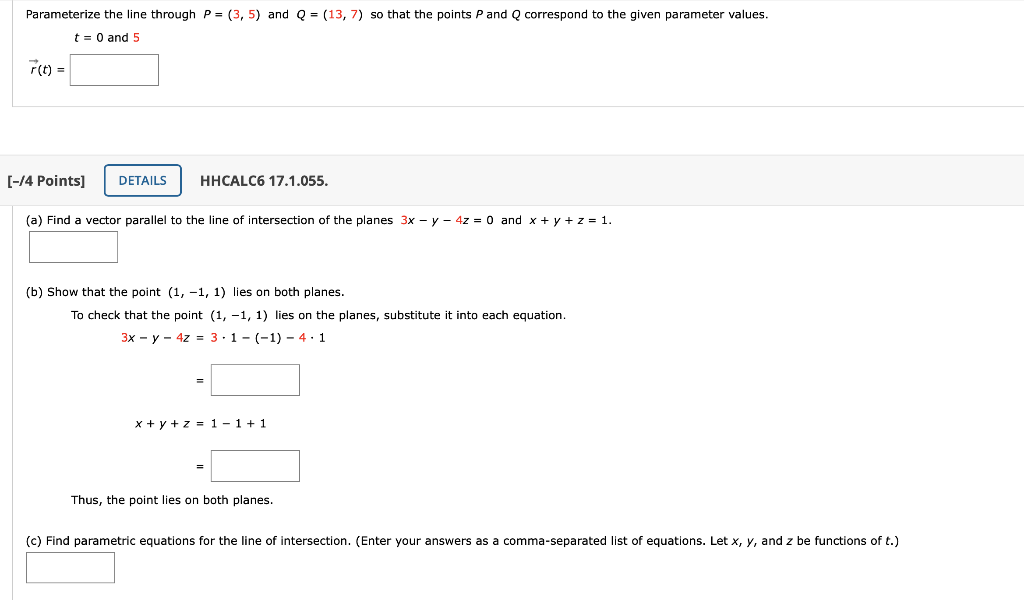# Question Parameterize the line through P = (3, 5) and Q = (13,7) so that the points P and Q correspond to the given parameter values. t = 0 and 5 (t) = [-14 Points] DETAILS HHCALCO 17.1.055. (a) Find a vector parallel to the line of intersection of the planes 3x - y - 4z = 0 and x + y + z = 1. (b) Show that the point (1,-1, 1) lies on both planes. To check that the point (1,-1, 1) lies on the planes, substitute it into each equation. 3x - y - 4z = 3.1- (-1) - 4.1 x + y + z = 1 - 1 + 1 Thus, the point lies on both planes. (c) Find parametric equations for the line of intersection. (Enter your answers as a comma-separated list of equations. Let x, y, and z be functions of t.)